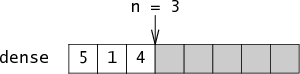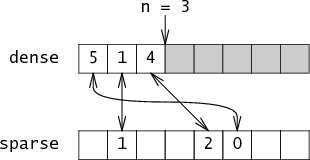# Marpa

fast sparse integer sets in CBounty program for improvements to Tcl and certain Tcl packages.

Up: Notes

References

Excerpts from the first reference:

• Preston Briggs and Linda Torczon's 1993 paper, “An Efficient Representation for Sparse Sets,” describes the trick in detail. Their solution represents the sparse set using an integer array named `dense` and an integer `n` that counts the number of elements in `dense`. The `dense` array is simply a packed list of the elements in the set, stored in order of insertion. If the set contains the elements 5, 1, and 4, then `n = 3` and `dense = 5`, `dense = 1`, `dense = 4`:• Together `n` and `dense` are enough information to reconstruct the set, but this representation is not very fast. To make it fast, Briggs and Torczon add a second array named `sparse` which maps integers to their indices in `dense`. Continuing the example, `sparse = 0`, `sparse = 1`, `sparse = 2`. Essentially, the set is a pair of arrays that point at each other:• To check whether `i` is in the set, you verify that the two arrays point at each other for that element.

• If `i` is not in the set, then it doesn't matter what sparse[i] is set to: either `sparse[i]` will be bigger than `n` or it will point at a value in `dense` that doesn't point back at it. Either way, we're not fooled.

An important part of this structure is that none of the memory it uses requires initialization before reading from it.